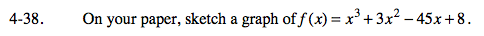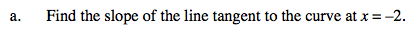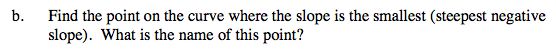### Home > CALC > Chapter 4 > Lesson 4.1.3 > Problem4-38

4-38.Recall that the slope of the tangent line at x = a is also known as f '(a).You are looking for the location where f '(x) is at its lowest (or at a minimum). To find this location, you will need to find the location (x-value) of the lowest point (the vertex) on the graph of f '(x). Note: f '(x) is a parabola, so an Algebra I student could complete this part of the task.

Use the x-value of the vertex of f '(x), to evaluate the coordinate point on f(x) where the slope is the steepest.